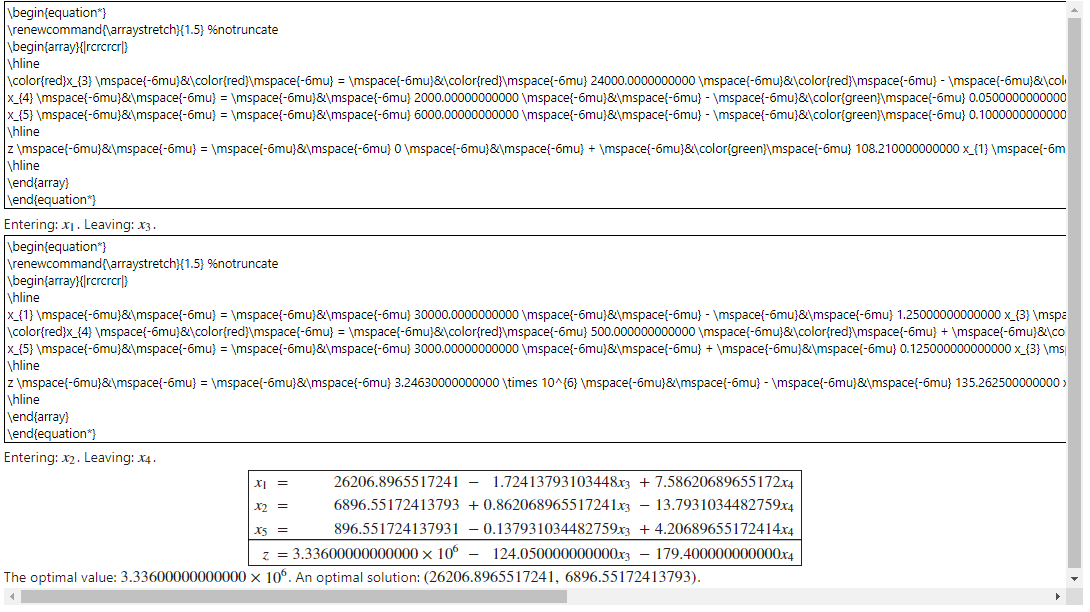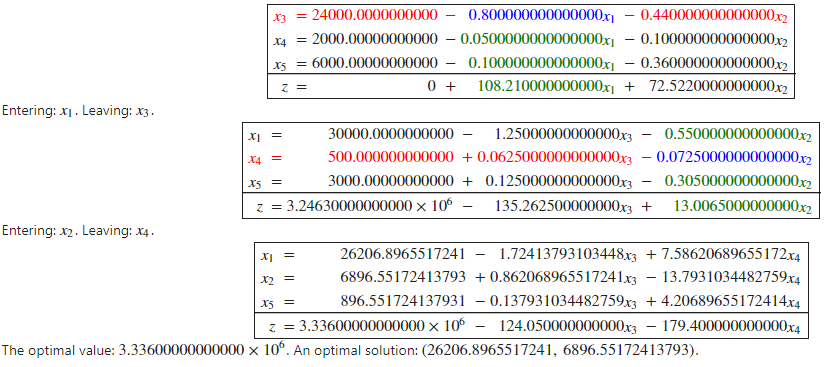# Displaying result problem

The results of the following code is perfectly displayed in QSagemath server but not in v 9.1 under windows 10

%display latex
A=[0.8,0.44],[0.05,0.1],[0.1,0.36]
b=(24000,2000,6000)
c=(108.21,72.522)
P=InteractiveLPProblemStandardForm(A,b,c,["x_1","x_2"],slack_variables=["e_3","e_4","e_5"])
P = P.standard_form()
P.run_simplex_method()
P = P.standard_form()
P.run_simplex_method()

edit retag close merge delete

• what is "QSagemath server"?
• what is the perfect display you see there and don't see elsewhere?
• under Windows10, are you using Sage with the Jupyter notebook?

Sort by » oldest newest most votedIt happens to me sometimes using Sagemath 9.1 in windows with jupyter-lab. I think Mathjax struggles when \color is not properly encapsulated within braces. here is a quick and dirty fix to remove all colors:

A=[0.8,0.44],[0.05,0.1],[0.1,0.36]
b=(24000,2000,6000)
c=(108.21,72.522)
P=InteractiveLPProblemStandardForm(A,b,c,["x_1","x_2"],slack_variables=["e_3","e_4","e_5"])
P = P.standard_form()
S = str(P.run_simplex_method())


then to display the result:

from IPython.display import Markdown, display
import re
S2 = re.sub(r"\\color{.*?}", "", S)
display(Markdown(S2))


It's possible to keep the colors, but I'm not knowledgeable enough in regex to do it with a one-liner, so it's even uglier:

from IPython.display import Markdown, display
import re

start = 0
S2 = ""
spans = [m.span() for m in re.finditer(r"\\color{.*?}", S)]
for s in spans:
end = min(S[s:].find('&'), S[s:].find(r'\\'))
S2 += S[start:s]+"{"+S[s:s]+"{"+S[s:s+end]+"}}"
start = s+end
S2 = S2+S[start:]
display(Markdown(S2))


Results: Before:After:more

Of course a real fix would be welcomed, but I have no idea where to start.